Electron. J. Diff. Eqns., Vol. 2009(2009), No. 78, pp. 1-19.

### On the rigidity of minimal mass solutions to the focusing mass-critical NLS for rough initial data Dong Li, Xiaoyi Zhang

Abstract:
For the focusing mass-critical nonlinear Schrodinger equation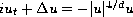, an important problem is to establish Liouville type results for solutions with ground state mass. Here the ground state is the positive solution to elliptic equation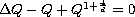. Previous results in this direction were established in [12, 16, 17, 29] and they all require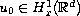. In this paper, we consider the rigidity results for rough initial data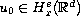for any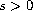. We show that in dimensions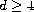and under the radial assumption, the only solution that does not scatter in both time directions (including the finite time blowup case) must be global and coincide with the solitary wave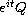up to symmetries of the equation. The proof relies on a non-uniform local iteration scheme, the refined estimate involving the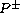operator and a new smoothing estimate for spherically symmetric solutions.

Submitted April 15, 2009. Published June 16, 2009.
Math Subject Classifications: 35Q55.
Key Words: Mass-critical; nonlinear Schrodinger equation.

Show me the PDF file (346 KB), TEX file, and other files for this article.

 Dong Li Institute for Advanced Study, Princeton, NJ, 08544, USA email: dongli@ias.edu Xiaoyi Zhang Academy of Mathematics and System Sciences, Beijing, China. Institute for Advanced Study, Princeton, NJ, 08544, USA email: xiaoyi@ias.edu